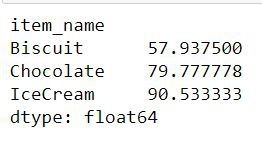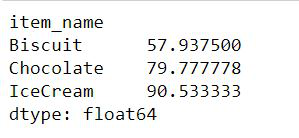# How to Calculate Weighted Average in Pandas?

• Last Updated : 09 Dec, 2021

A weighted average is a computation that considers the relative value of the integers in a data collection. Each value in the data set is scaled by a predefined weight before the final computation is completed when computing a weighted average.

Syntax:

```def weighted_average(dataframe, value, weight):
val = dataframe[value]
wt = dataframe[weight]
return (val * wt).sum() / wt.sum()```

It will return the weighted average of the item in value. In the numerator, we multiply each value with the corresponding weight associated and add them all. In the denominator, all the weights are added.

### Approach

• We take a data frame or make our own data frame.
• Define a function to calculate the weighted average by the above-mentioned formula.
• We need to have at least three items in the data frame i.e index (which may be item-name, date, or any such variable), value, and weight.
• We will make a function call passing all these three values.

Example:

Let us see an example to calculate the weighted average of value grouped by item_name.

Suppose there are three shops and each shop contains three items i.e Chocolates, IceCream and Biscuits. We have the weight of each of the items and the price of each of the items in all the three shops. Now we need to find out the weighted average of each item.

## Python3

 `import` `pandas as pd`  `def` `weighted_average(dataframe, value, weight):``    ``val ``=` `dataframe[value]``    ``wt ``=` `dataframe[weight]``    ``return` `(val ``*` `wt).``sum``() ``/` `wt.``sum``()`  `# creating a dataframe to represent different``# items and their corresponding weight and value``dataframe ``=` `pd.DataFrame({``'item_name'``: [``'Chocolate'``, ``'Chocolate'``,``                                        ``'Chocolate'``, ``'Biscuit'``,``                                        ``'Biscuit'``, ``'Biscuit'``,``                                        ``'IceCream'``, ``'IceCream'``,``                                        ``'IceCream'``],``                          ``'value'``: [``90``, ``50``, ``86``, ``87``, ``42``, ``48``,``                                    ``68``, ``92``, ``102``],``                          ``'weight'``: [``4``, ``2``, ``3``, ``5``, ``6``, ``5``, ``3``, ``7``,``                                     ``5``]})` `# Weighted average of value  grouped by item name``dataframe.groupby(``'item_name'``).``apply``(weighted_average,``                                     ``'value'``, ``'weight'``)`

Output:## Using groupby()

Here we are going to group the items using groupby() function and calculate the weights by grouping these items along with sum function. So By using this method we are just forming a group of  similar items to get the sum

Syntax :

```def weighted_average_of_group(values, weights, item):
return (values * weights).groupby(item).sum() / weights.groupby(item).sum()```

Example:

## Python3

 `import` `pandas as pd`  `def` `weighted_average_of_group(values, weights, item):``    ``return` `(values ``*` `weights).groupby(item).``sum``() ``/` `weights.groupby(item).``sum``()`  `# creating a dataframe to represent different items``# and their corresponding weight and value``dataframe ``=` `pd.DataFrame({``'item_name'``: [``'Chocolate'``, ``'Chocolate'``, ``'Chocolate'``,``                                        ``'Biscuit'``, ``'Biscuit'``, ``'Biscuit'``,``                                        ``'IceCream'``, ``'IceCream'``, ``'IceCream'``],``                          ``'value'``: [``90``, ``50``, ``86``, ``87``, ``42``, ``48``, ``68``, ``92``, ``102``],``                          ``'weight'``: [``4``, ``2``, ``3``, ``5``, ``6``, ``5``, ``3``, ``7``, ``5``]})` `# Finding grouped average of group``weighted_average_of_group(values``=``dataframe.value,``                          ``weights``=``dataframe.weight, item``=``dataframe.item_name)`

Output:To calculate the weighted average of the whole data frame (not of every group, but as a whole) we will use the syntax shown below:

Syntax

```def weighted_average_of_whole_dataframe(dataframe, value, weight):
val = dataframe[value]
wt = dataframe[weight]
return (val * wt).sum() / wt.sum()```

Example:

## Python3

 `import` `pandas as pd`  `def` `weighted_average(dataframe, value, weight):``    ``val ``=` `dataframe[value]``    ``wt ``=` `dataframe[weight]``    ``return` `(val ``*` `wt).``sum``() ``/` `wt.``sum``()`  `# creating a dataframe to represent different items``# and their corresponding weight and value``dataframe ``=` `pd.DataFrame({``'item_name'``: [``'Chocolate'``, ``'Chocolate'``, ``'Chocolate'``,``                                        ``'Biscuit'``, ``'Biscuit'``, ``'Biscuit'``,``                                        ``'IceCream'``, ``'IceCream'``, ``'IceCream'``],``                          ``'value'``: [``90``, ``50``, ``86``, ``87``, ``42``, ``48``, ``68``, ``92``, ``102``],``                          ``'weight'``: [``4``, ``2``, ``3``, ``5``, ``6``, ``5``, ``3``, ``7``, ``5``]})` `# Weighted average of whole dataframe as a whole``weighted_average(dataframe, ``'value'``, ``'weight'``)`

Output:

`75.075`

My Personal Notes arrow_drop_up